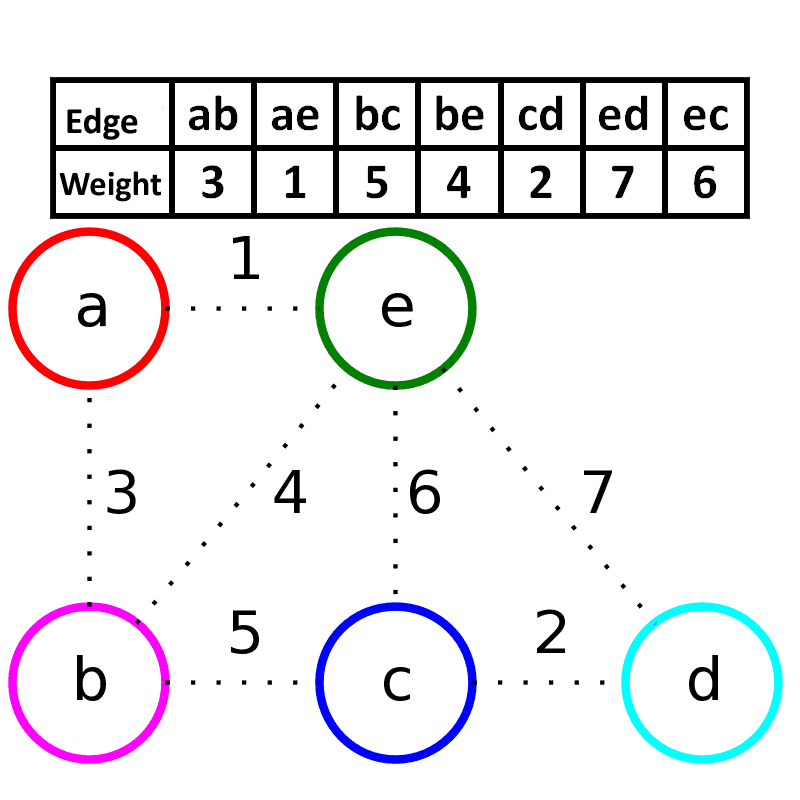# 图论 - 最小生成树与 Kruskal 算法

## 最小生成树

### 树与生成树

$$w(T) = \sum_{(u, v) \in T} w(u, v)$$

#### 特殊性质

$$|E| = v - 1$$

（其实我也是看 Wikipedia 上的嘿嘿😁）

## Kruskal 算法

1. 首先将图 $G(V, E)$ 的边集合拿出按照权值进行排序
2. 之后从最小权值的边开始不断 “回填”
3. 在回填过程中如果发现形成了环，则跳过这条边的回填
4. 当回填的边数量达到 $v - 1$ 时停止，其中 $v$ 是原图顶点集合的势